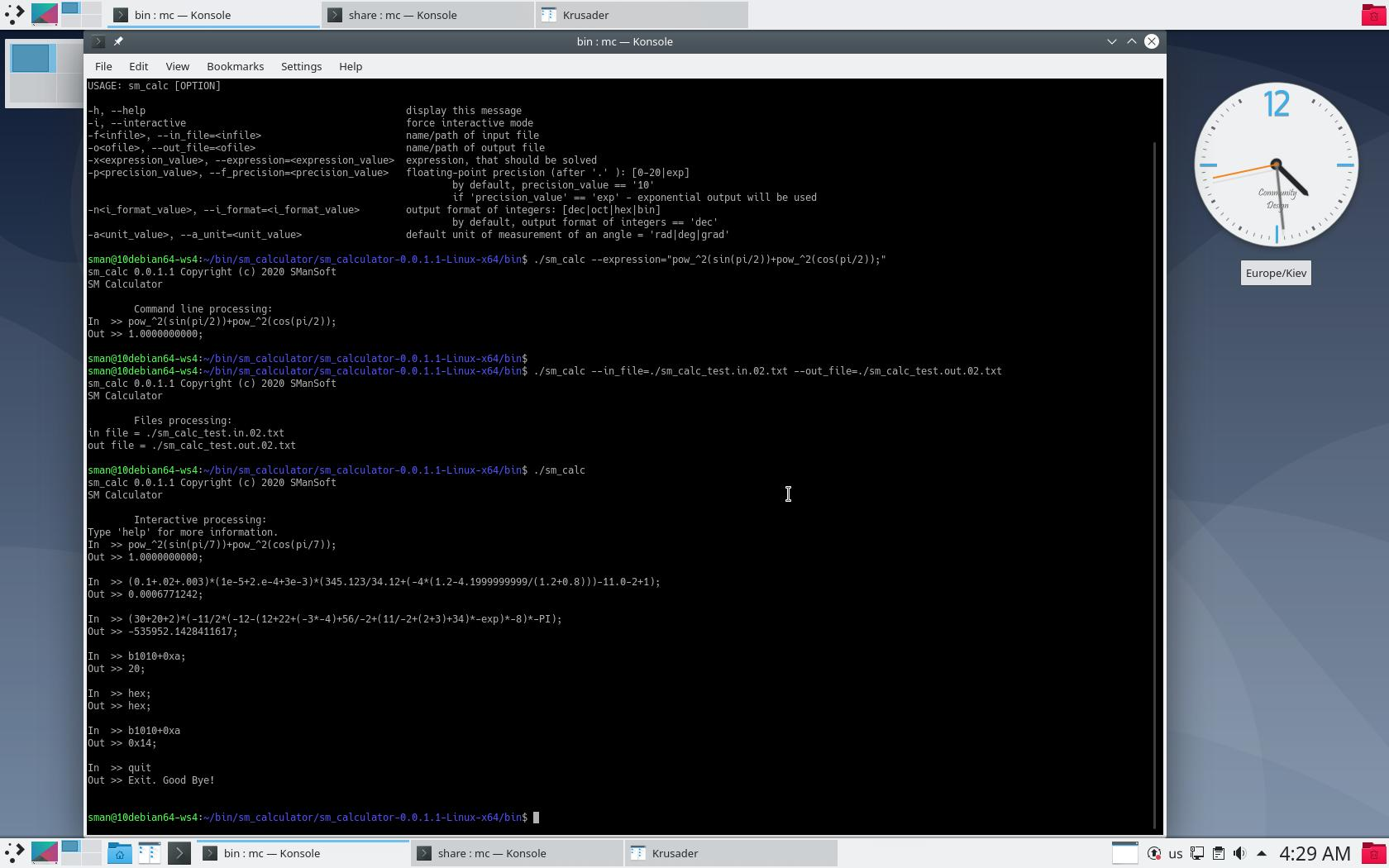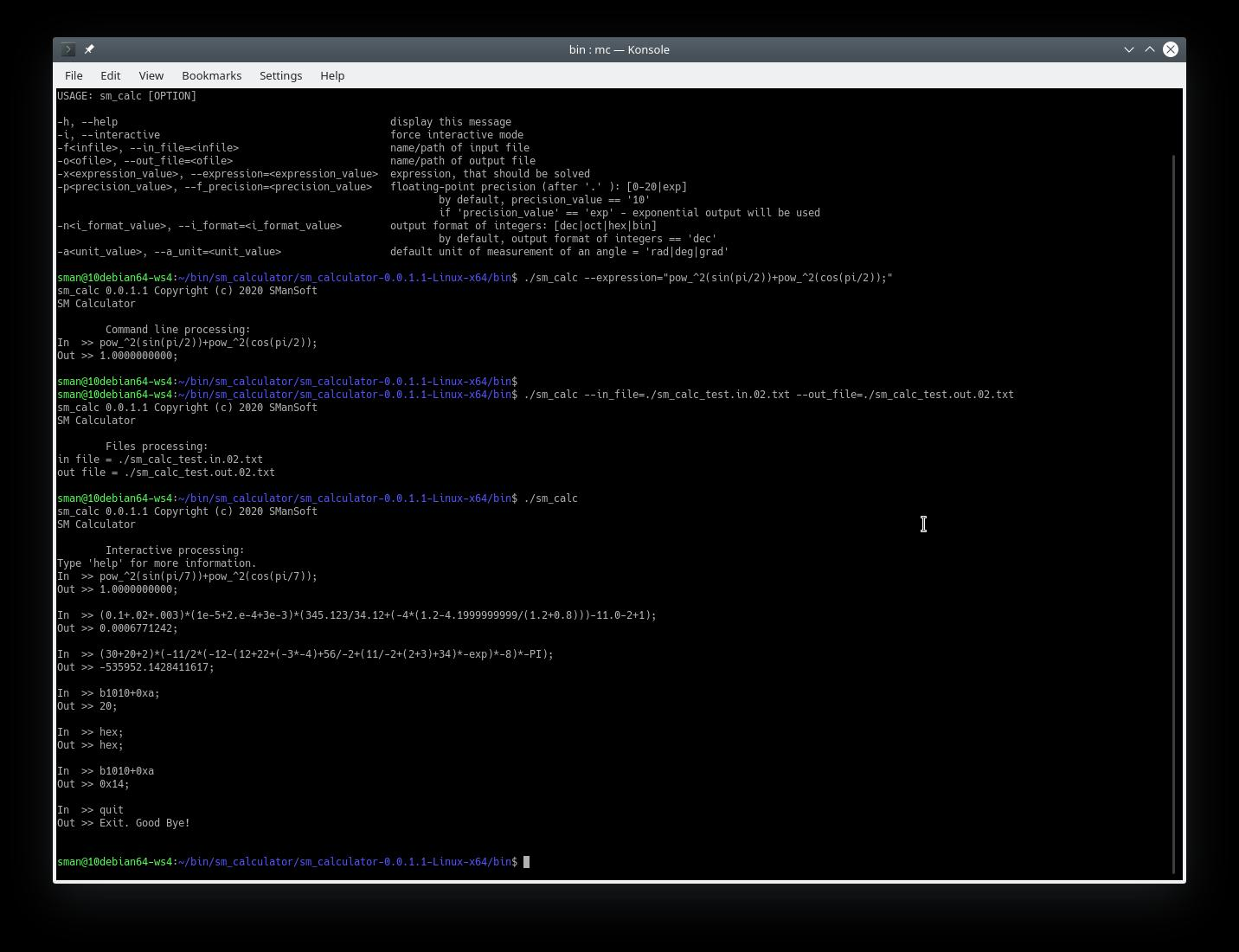# Multi-Platform Open-Source sm_calculator (SM Calculator) tool 0.1.1.3

Multi-Platform Open-Source sm_calculator (SM Calculator) tool 0.1.1.3 has been released.

New features of the SM Calculator (0.1.1.3):
—————-

• Detailed processing of syntax error(s);
• Restoring of syntax parsing after error(s);

Supported platforms:

• Windows (x86, x86_64)
• Linux (x86, x86_64)
• Examples, the suite of sm_calc_test.in.XX.txt and sm_calc_test.out.XX.txt (also you can find the suite of sm_calc_test.in.XX.txt and sm_calc_test.out.XX.txt in directory /tests in sources):
Examples

—————-

GPG Public Key (SManSoft ECDSA Key) <info@smansoft.com> has been published here: http://smansoft.com/gpg/smansoft.pub.asc.
SM Calculator – 0.1.1.3 contains suite of [some_install_file].asc files.
`gpg --import ./smansoft.pub.asc`
for importing of key and
`gpg --verify ./[some_install_file].asc ./[some_install_file]`
or
`gpg --verify ./[some_install_file].asc`
for verification of files, published on this site.
Public GPG ECDSA Key can be imported, using
`gpg --keyserver hkp://pgp.mit.edu --recv-keys A408B0FCFD774649`
too.

Features of the sm_calculator (SM Calculator) tool 0.1.1.3:
—————-

• Multi-platform application: there are builds for Windows 64/32 (.zip) and Linux 64/32 (.tar.gz);
• Various types of values (64 bits integers, 64 bits floats, strings);
• Various float constants (pi, exp,…);
• Solving of mathematical expressions, using basic arithmetic operators;
• Solving of mathematical expressions, using bracket delimiters ‘(‘ and ‘)’ (in one expression) and delimiters ‘;’, ‘\n’;
• Solving using suite of mathematical (including trigonometric, logarithm, exponentiation) functions;
• Solving using bitwise functions and operators;
• Various types of entering and output formats of integers (decimal, octal, hexadecimal, binary formats);
• Various values of the unit for measuring of angles (radians, degrees, gradians), which are used by trigonometric functions;
• Support of output properties (precision) of float values;
• The suite of converting functions (various values of the unit for measuring of angles, various types of entering and output formats of integers);
• the suite of commands, which provide setup of the configuration of SM Calculator;
• Several modes of execution (processing of files, processing of expressions, obtained via command line, processing of expressions in interactive mode, using standard input/output devices);
• Control/administration the creation/start of the log of the sm_calculator (SM Calculator) tool:
command-line option: `-q, --no_log`
– changing the path of the created/opened log file:
command-line option: `-l, --log_file`;
• Access to the sm_calculator (SM Calculator) tool can be included into \$PATH (Windows/Linux) and the sm_calculator (SM Calculator) tool can be launched from any current directory;
• Detailed processing of syntax error(s);
• Restoring of syntax parsing after error(s);
• Help:
– command: `help[;]`
– command-line option: `-h, --help;`

SM Calculator tool supports the following modes of execution:
—————-

• processing of the file, that contains expression(s);
• processing of command-line expression(s);
• interactive mode (user enters expression(s), using standard input device/console).

A user can use the same expressions in all these modes (processing of the file,
processing of command-line expression(s), interactive mode). I.e. SM Calculator tool supports the same lexemes and grammar rules in all modes.

Limitations/disadvantages of the sm_calculator (SM Calculator) tool 0.1.1.3:
—————-

• sm_calculator (SM Calculator) tool 0.1.1.3  writes full log with detailed info without filtering of log messages by log level and without rolling of log files; So, a user should control the length of the log file by himself;
• sm_calculator (SM Calculator) tool 0.1.1.3 doesn’t guarantee correct execution when a user uses Unicode (UTF-16, partially UTF-8) encoding; sm_calculator (SM Calculator) tool 0.1.1.3 has been tested, for the usage of US-ASCII encoding symbols; Possible (according to cursory testing) user can use UTF-8 encoding (including national symbols) on Linux platform;

Structure of binary archive (.zip or .tar.gz):
—————-

• `.zip (Windows):`
```    bin\
sm_calc.exe
share\smansoft\doc\
HELP
CHANGELOG
```
• `.tar.gz (Linux):`
```    bin/
sm_calc
lib/
libgetopt_lib.so
share/smansoft/doc/
HELP
CHANGELOG
```

Execution:
—————-
`sm_calc[.exe]`
without parameters launches SM Calculator in interactive mode (default mode):

``````sm_calc 0.1.1.3 Copyright (c) 2020 SManSoft
Log has been started in the file: <path-to-log-dir>/sm_calc.log
SM Calculator
``` ```    Interactive processing:
In >>``````

User can run:
`sm_calc[.exe] -h`
or
`sm_calc[.exe] --help`
to get help output – description of command-line parameters.

Using these options a user can launch SM Calculator in various modes of execution and tune start values of the current configuration of SM Calculator (command: config[;]):

```-h, --help -i, --interactive -l<lfile>, --log_file=<lfile> -q, --no_log -f<infile>, --in_file=<infile> -o<ofile>, --out_file=<ofile> -x<expression_value>, --expression=<expression_value> -p<precision_value>, --f_precision=<precision_value> -n<i_format_value>, --i_format=<i_format_value> -a<unit_value>, --a_unit=<unit_value>```

Lexemes of SM Calculator expressions:
—————-

Available keywords:
`f_precision i_format a_unit pi exp gamma phi rad deg grad grad2rad rad2grad rad2deg deg2rad grad2deg deg2grad dec oct hex bin dec2oct dec2hex dec2bin oct2dec hex2dec bin2dec sin cos tan asin acos atan ln lg log inv sqrt rand pow pow_exp^ pow_10^ pow_2^ pow_^2 abs mod mod2 and or not nand nor xor config help echo quit exit`

Available operators:
`+ - * / = ! <a< >a> <l< >l> <c< >c>`

Available delimiters:
`, ( ) ; \n (new line)`

Commands (from available keywords):
`f_precision i_format a_unit dec oct hex bin rad deg grad rand config help quit exit`

Constants (from available keywords):
`pi exp gamma phi`

Output functions (from available keywords):
`echo`

Converting functions (from available keywords):
`grad2rad rad2grad rad2deg deg2rad grad2deg deg2grad dec2oct dec2hex dec2bin oct2dec hex2dec bin2dec`

Trigonometric functions (from available keywords):
`sin cos tan asin acos atan`

Logarithm functions (from available keywords):
`ln lg log`

Exponentiation functions (from available keywords):
`pow pow_exp^ pow_10^ pow_2^ pow_^2`

Bit functions (from available keywords):
`and or not nand nor xor`

Bit operators (from available operators):
`<a< >a> <l< >l> <c< >c>`

Base syntax of commands:
—————-
`command[;]`
`command=arg[;]`

Base syntax of functions:
—————-
`function (arg1[,arg2])[;]`

Base syntax of operators:
—————-
`arg1 operator arg1[;]`
`operator arg[;]`

Operator assign (‘=’) can be used only in commands (f_precision, i_format, a_unit).

Types of values:
—————-

• Integer data (long long type – 64 bits);
• Float data (long double type – 64 bits);
• Strings (max length of string 1021 ASCII symbols);

Integer data:
—————-
Length of Integer data – 64 bits (including sign), i.e.
max integer value == 2^63-1;
min integer value == -(2^63-1);
Integer value can be entered and output in follow formats:

• decimal (‘dec‘);
• octal (‘oct‘);
• binary (‘bin‘);

The output format of integer value can be defined by command:
`i_format = <dec|hex|oct|bin>[;]`
A user can use shorter way for defining of the output format of an integer value, using commands:
`dec[;]`
`hex[;]`
`oct[;]`
`bin[;]`

The input format of an integer is defined by following rules:

• if a user enters integer value in octal format, he should use as least one lead ‘0’ symbol (for example: ‘0712377’, ‘-0712377’);
• if user enters integer value in hexadecimal format, he should use as lead ‘0x’ symbols (for example: ‘0x394ff’, ‘0xfffffffffffc6b01’);
• if user enters integer value in binary format, he should use as lead ‘b’ symbol (for example:  ‘b00111001010011111111’‘b1111111111111111111111111111111111111111111111000110101100000001’);
• if user enters integer value in decimal format, he shouldn’t use any additional symbols (for example: ‘129077’‘-129077’);

A user should take into account, that size of integer value is 64 bits (including sign), during entering value in various formats;

A user can enter negative values (in octal and hexadecimal) using as sign ‘-‘
as two’s complement format (see examples in the file ReadMe.txt):
-0712377 (oct) or 01777777777777777065401 (oct) == -234751 (dec)
-0x394ff (hex) or 0xfffffffffffc6b01 (hex) == -234751 (dec)

If user need to get current output format of integer values, he needs to use command:
`i_format[;]`

SM Calculator tool supports the suite of functions, which provide converting of various formats of integer values, whatever defined current output format of integer values:
`dec2oct dec2hex dec2bin oct2dec hex2dec bin2dec`.

Float data (long double – 64 bits):
—————-
The output format of float values always defined in decimal format. User can tune the precision of float value output, using the command:
`f_precision = N[;]`
, where N = [0,20]|exp, i.e. maximum precision of output of float value is ’20’.

Also, N can be defined as ‘exp’. In this case, the float value will be printed in an exponential format.

The input format of float values always defined in decimal format. A user can enter values as in floating form format as in exponential format.

If a user needs to get current output current precision of float, he needs to use the command:
`f_precision[;]`.

Strings:
—————-
User should enter string value, using the following format:
`"string_value" | 'string_value'`.
Max length of the string – 1023 ASCII symbols with quotes(‘ or “)
or 1021 ASCII symbols without quotes(‘ or “).

String values aren’t used during calculations but could be used for the formatting of outputs in file processing, using the function
`echo`.

Default angle unit configuration:
—————-
SM calculator supports some suite of trigonometrical functions:
`sin cos tan asin acos atan`.
Also, SM calculator supports follow units for measuring of angles:

• degrees(‘deg‘)

SM Calculator tool contains the current configuration of the unit for measuring of angles. This parameter defines, which unit for measuring of angles will be used by trigonometric functions, i.e. if the current configuration of the unit for measuring of angles is radians, a user should use radians as parameters of trigonometric functions: sin(3.1415926536) or sin(pi), cos(1.5707963268) or cos(pi/2.0), if the current configuration of the unit for measuring of angles is degrees, a user should use degrees as parameters of trigonometric functions: sin(180.0), cos(90), same issue with the usage of gradians.
The current configuration of the unit for measuring of angles can be defined by the following command:
`a_unit=<deg|rad|grad>[;]`

Also, user can setup current configuration of the unit for measuring of angles, just using following commands:
`deg[;]`
`rad[;]`
`grad[;]`

User can get current configuration of the unit for measuring of angles using the command:
`a_unit[;]`

SM Calculator tool supports the suite of functions, which provide converting of the various unit for measuring of angles, whatever defined current configuration of the unit for measuring:

`grad2rad rad2grad rad2deg deg2rad grad2deg deg2grad`

Current configuration:
—————-
A user always can get current configuration, using the command:
`config[;]`
The result of the command config[;]:
```Current Configuration : --------------------------------------------- Current double precision : EXPONENTIAL; Current output format of integers : dec; Unit of measurement of an angle : rad;```

Constants:
—————-
SM Calculator supports follow float (64 bits) constants:
`pi`
`exp`
`gamma`
`phi`

Constants return float values. They can be used in expressions as arguments of functions and operators.

Examples:
—————-
User can find here suite of files which contain suite examples of usage of operators, functions, commands,
constants, various types of data:

Examples

 Examples of expressions: Results of execution of expressions: sm_calc_test.in.01.txt sm_calc_test.out.01.txt sm_calc_test.in.02.txt sm_calc_test.out.02.txt sm_calc_test.in.03.txt sm_calc_test.out.03.txt sm_calc_test.in.04.txt sm_calc_test.out.04.txt sm_calc_test.in.05.txt sm_calc_test.out.05.txt sm_calc_test.in.06.txt sm_calc_test.out.06.txt sm_calc_test.in.07.txt sm_calc_test.out.07.txt sm_calc_test.in.08.txt sm_calc_test.out.08.txt

Also, Examples contains:

• screenshots (.jpeg);
• video demo (.mp4);
• demo log files (.log);

—————-

Help.txt:
—————-
The short reference of commands, constants, functions, operators of the sm_calculator (SM Calculator) tool 0.1.1.3 is published here:
Help.txt

Demo of the sm_calculator (SM Calculator) tool 0.1.1.3 (Windows):
—————-

Here are the results of the execution of the sm_calculator (SM Calculator) tool 0.1.1.3 on Microsoft Windows 10 x64.

The sm_calculator (SM Calculator) tool 0.1.1.3 has been launched 4 times:

1. `sm_calc --help`
2. `sm_calc --expression="pow_^2(sin(pi/2))+pow_^2(cos(pi/2));"`
3. `sm_calc --in_file="./sm_calc_test.in.01.txt" --out_file="./sm_calc_test.out.01.txt"`
4. `sm_calc`

Results of execution (log file and screenshots) below:
sm_calc.log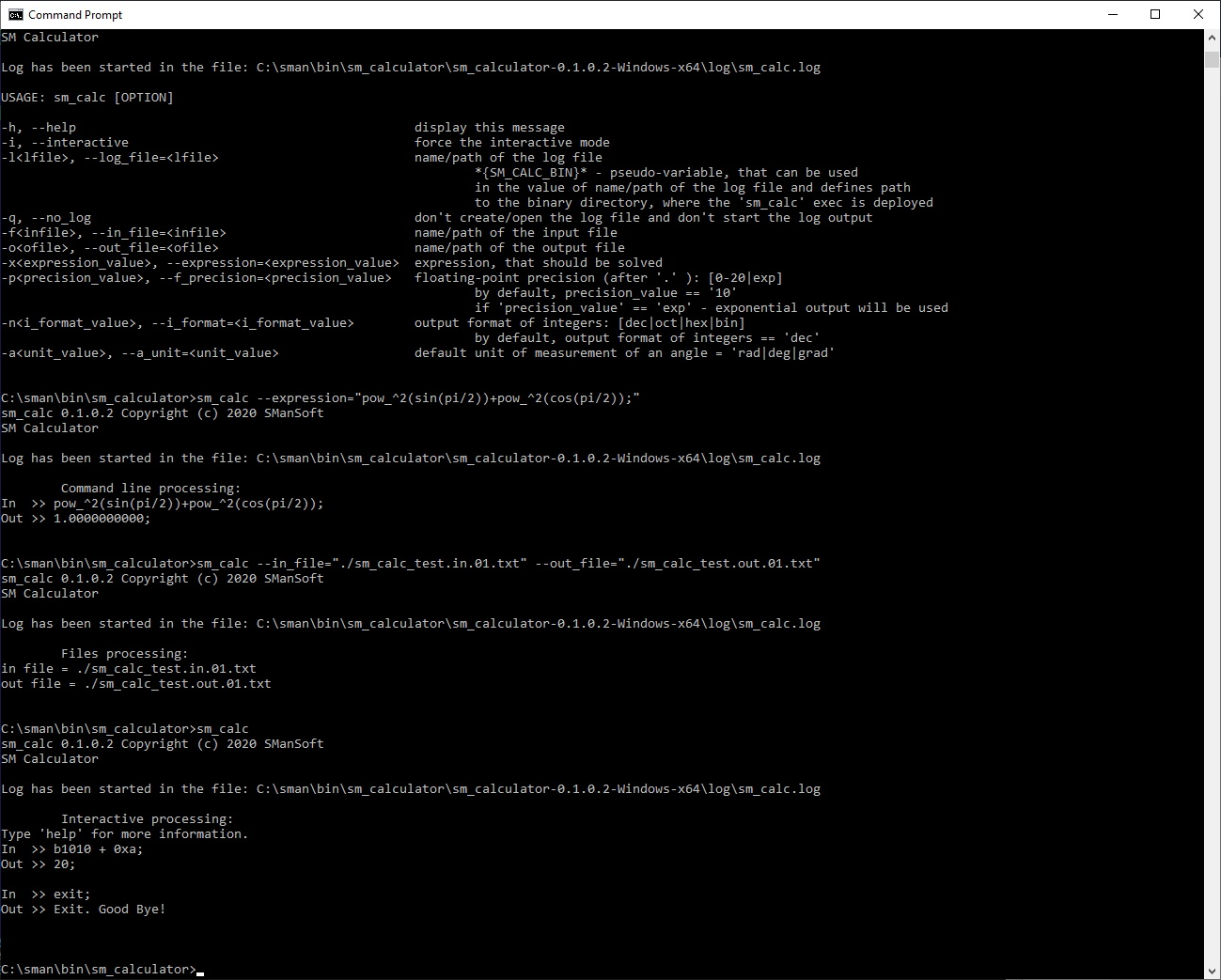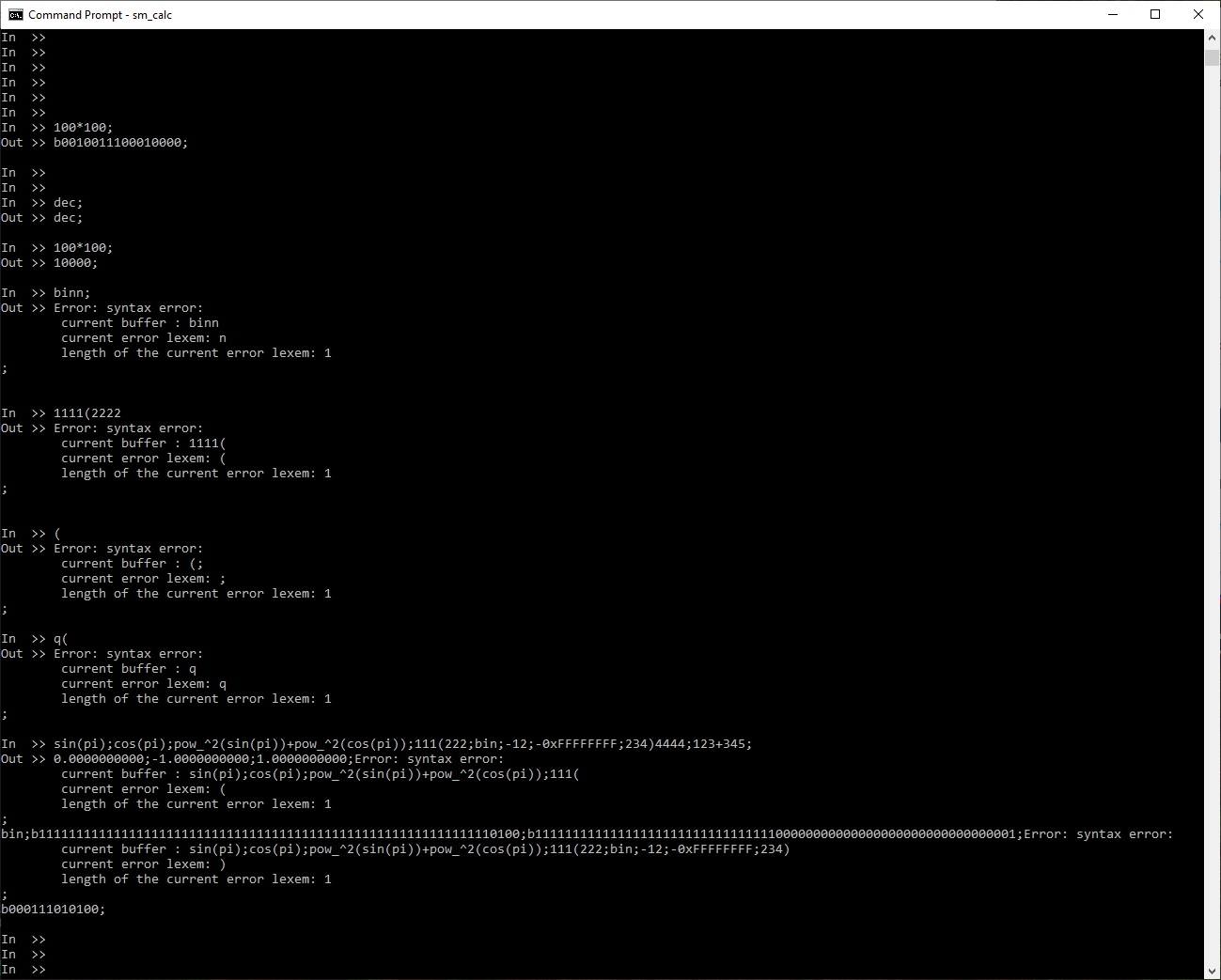Demo of the sm_calculator (SM Calculator) tool 0.1.1.3 (Linux):
—————-

Here are the results of the execution of the sm_calculator (SM Calculator) tool 0.1.1.3 on Linux Debian 10 (Buster) x64.

The sm_calculator (SM Calculator) tool 0.1.1.3 has been launched 4 times:

1. `sm_calc --help`
2. `sm_calc --expression="pow_^2(sin(pi/2))+pow_^2(cos(pi/2));"`
3. `sm_calc --in_file="./sm_calc_test.in.02.txt" --out_file="./sm_calc_test.out.02.txt"`
4. `sm_calc`

Results of execution (log file and screenshots) below:
sm_calc.log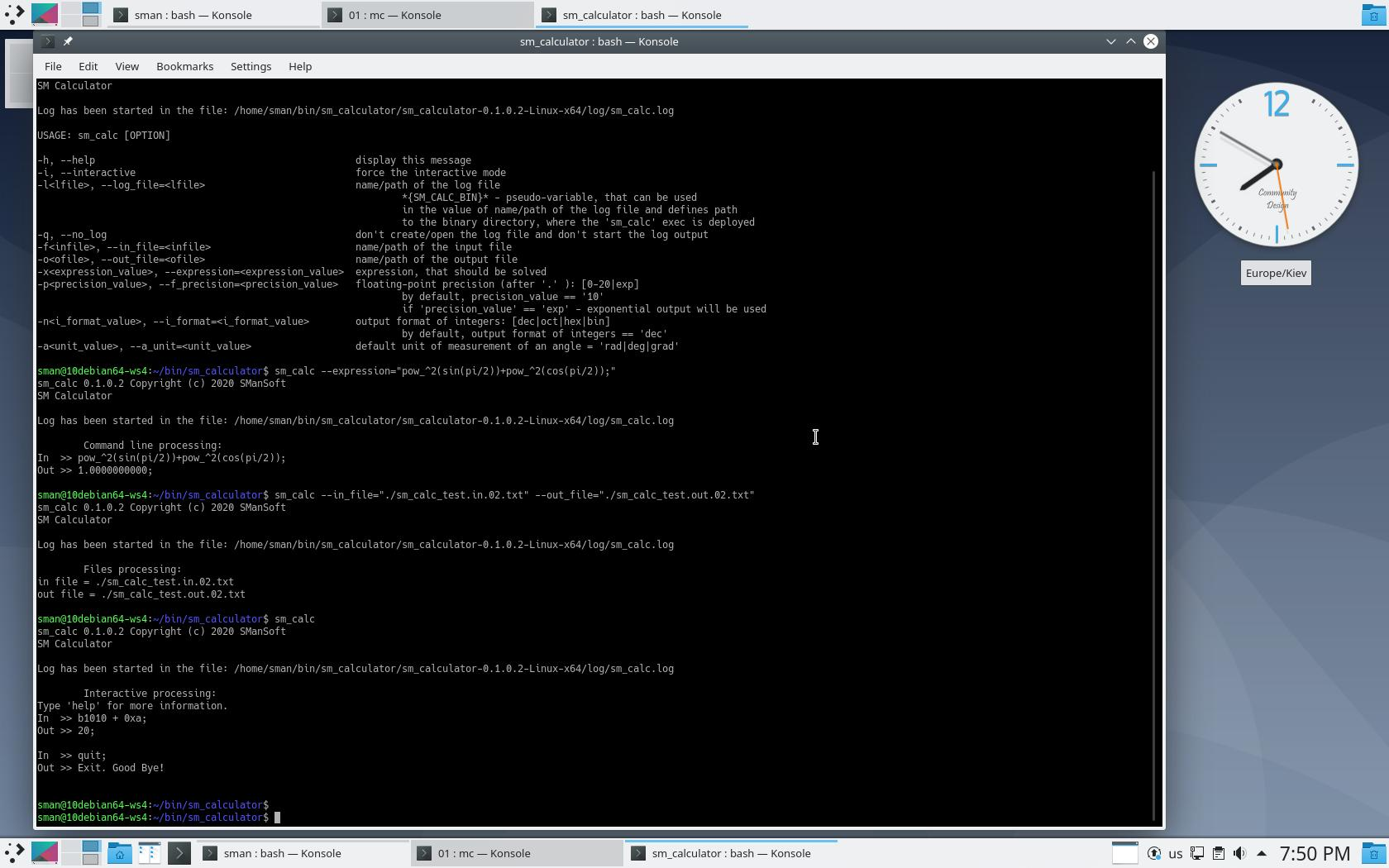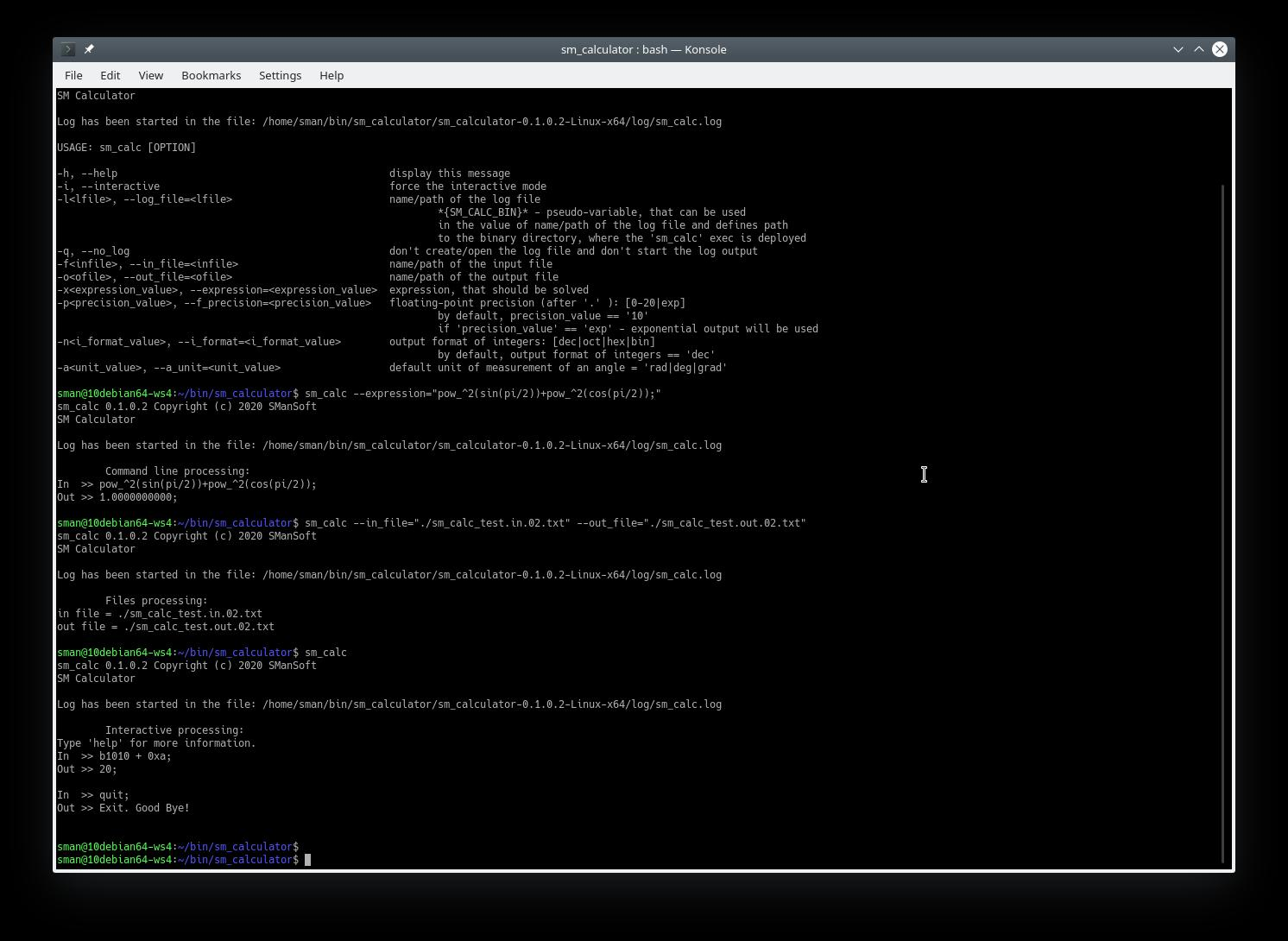—————-

# Building the sm_calculator (SM Calculator) solution 0.1.0.2

Description of sources, structure, third-party tools, and assembling the Multi-Platform Open-Source sm_calculator (SM Calculator) solution 0.1.0.2.

—————-

Source files of the sm_calculator (SM Calculator)
can be found here:
SM Calculator – 0.1.0.2
sm_calculator-0.1.0.2-Source.zip
sm_calculator-0.1.0.2-Source.tar.gz

Also, you can find source files here:
sm_calculator on GitHub

You can use the command:
`git clone https://github.com/smansoft/sm_calculator.git`
to get sources from the GitHub repository.

Install.txt
—————-
Here is a detailed description of the solution, sources, necessary tools, and ways, how the sm_calculator (SM Calculator) solution 0.1.0.2 can be built and tested:
Install.txt.

Third-party libraries and tools
—————-
You can find a suite of third-party libraries and tools here (SM Calculator – 0.1.0.2): cmocka, getopt, safestringlib, win_flex_bison (Install.txt). Here are:

• win_flex_bison – windows port of Flex and Bison tools
• getopt – library (adopted for Visual Studio) for parsing of command-line aruments
• safestringlib – a multi-platform library, that contains API for safe control of memory and strings
• cmocka – unit test library

You can download win_flex_bison for assembling the sm_calculator (SM Calculator) solution 0.1.0.2 on Windows and you don’t need other third-party libraries for assembling the sm_calculator (SM Calculator) solution 0.1.0.2 as they are already included in sources (/deps).

Tools necessary for assembling of the project:
—————-

Windows platform:

• Visual Studio 2019 (including NMake tool);
• CMake;
• flex;
• bison;
• diff (optionally);
• Python 3 (optionally);

Linux platform:

• GCC;
• Make tool;
• CMake;
• flex;
• bison;
• bash;
• diff (optionally);
• Python 3 (optionally);

Some issue of assembling on the Linux platform, using synchronized log (definition: SM_SYNC_LOG):
—————-

The sm_calculator (SM Calculator) solution 0.1.0.2 contains project log_lib, which contains the support of synchronized print (definition: SM_SYNC_LOG). As a rule, there is no necessity of usage of synchronizing print, as every log print is executed by one call of runtime the function `fprintf`  of synchronized C-runtime API. But there are some cases when this synchronizing can de used (Install.txt). Windows tree of sources uses standard critical section objects in synchronizing API. Linux tree of sources uses thread API, defined by C11 (ISO/IEC 9899:2011) standard, using `#include <threads.h>`. But not all packages of GCC contain the support of the thread API, defined by C11 (threads.h), though GCC supports the C11 standard. This problem can be resolved after updating of usage of the thread API, defined by C11 (`#include <threads.h>`) to the usage of the POSIX thread API (`#include <pthread.h>`).

Build of the sm_calculator (SM Calculator) solution 0.1.0.2 (Windows):
—————-

Here is a build screenshot, using
`/_cmake/cmake_build.nmake.bat`
See Install.txt for more details about the way of a build of the solution, using `/_cmake/cmake_build.nmake.bat`. /_cmake – means subdirectory _cmake of the root of sources of the sm_calculator (SM Calculator) solution 0.1.0.2.

Build of the sm_calculator (SM Calculator) solution 0.1.0.2 includes following steps:

• creating of the subdirectory /_cmake/_build
• `cmake -G "NMake Makefiles" -DCMAKE_BUILD_TYPE="Release" "..\.."` – generating of necessary project/build files, including Makefile for the NMake tool
• `nmake clean` – clean target in Makefile (generated by CMake)
• `nmake` – build of the solution
• `nmake test` – launching unit tests
• `cpack -G "ZIP"` – generating pack archive, that contains binaries (.zip)
• `cpack -G "ZIP" --config CPackSourceConfig.cmake` – generating pack archive, that contains sources (.zip)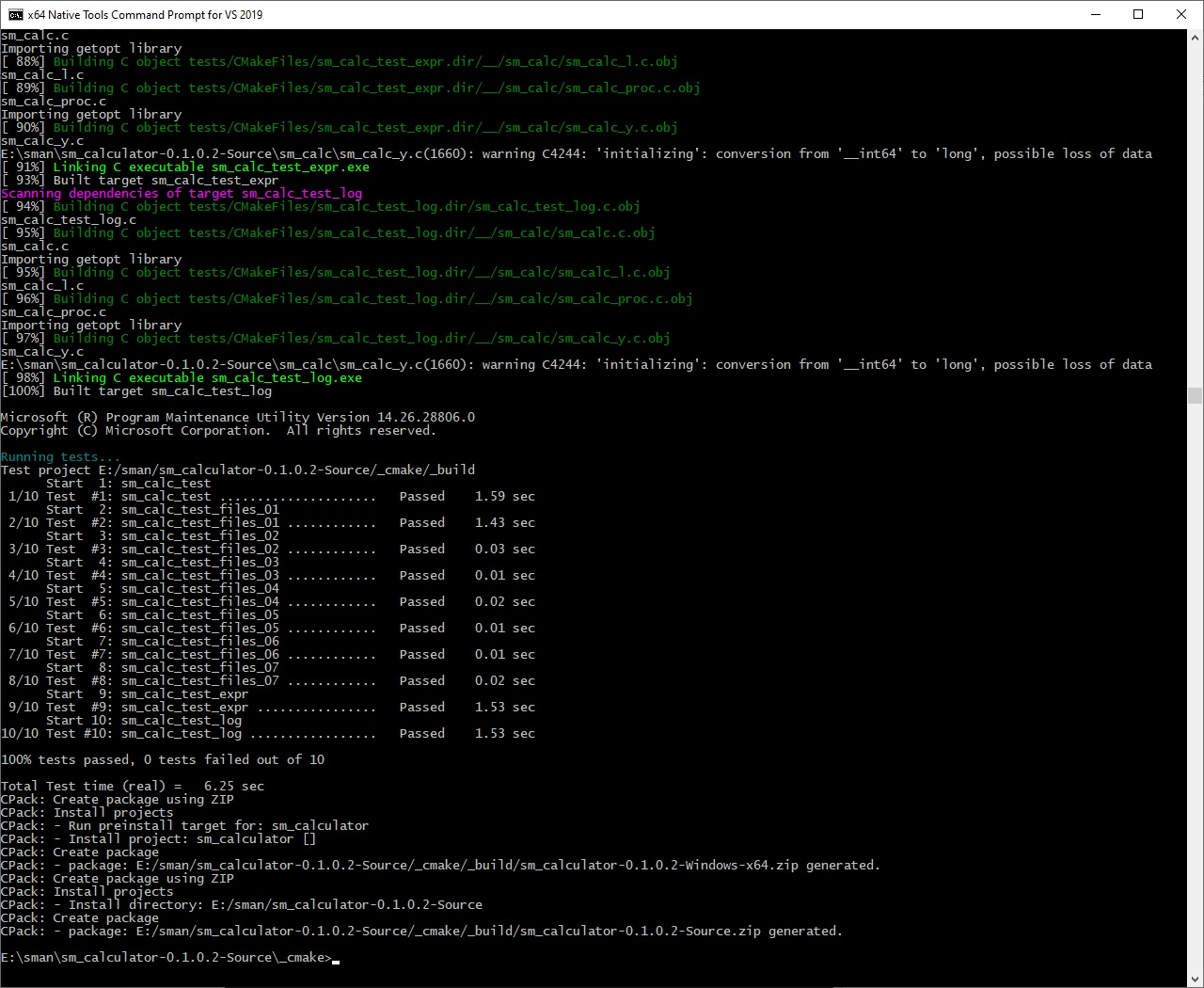Build of the sm_calculator (SM Calculator) solution 0.1.0.2 (Linux):
—————-

Here is a build screenshot, using
`/_cmake/cmake_build.sh`
See Install.txt for more details about the way of a build of the solution, using `/_cmake/cmake_build.sh`.

Build of the sm_calculator (SM Calculator) solution 0.1.0.2 includes following steps:

• creating of the subdirectory /_cmake/_build
• `cmake -G "Unix Makefiles" -DCMAKE_BUILD_TYPE="Release" "../..""` – generating of necessary project/build files, including Makefile for the Make tool
• `make clean` – clean target in Makefile (generated by CMake)
• `make all test` – build of the solution and launching unit tests
• `cpack -G "TGZ"` – generating pack archive, that contains binaries (.tar.gz)
• `cpack -G "TGZ" --config CPackSourceConfig.cmake` – generating pack archive, that contains sources (.tar.gz)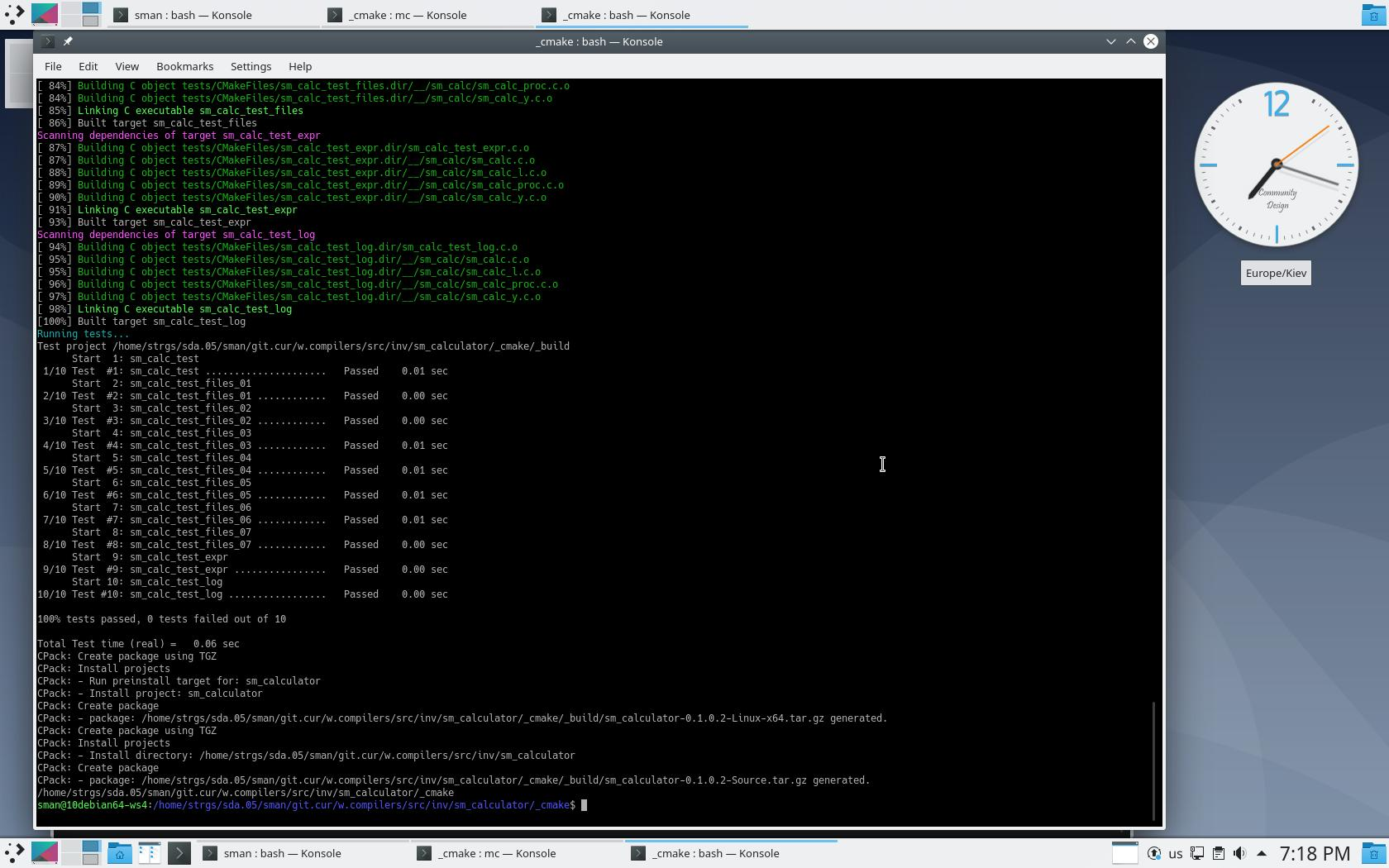—————-

# Multi-Platform Open-Source sm_calculator (SM Calculator) tool 0.1.0.2

Multi-Platform Open-Source sm_calculator (SM Calculator) tool 0.1.0.2 has been released.

New features of the SM Calculator (0.1.0.2):
—————-

• Control/administration the creation/start of the log of the sm_calculator (SM Calculator) tool:
command-line option: `-q, --no_log`
– changing the path of the created/opened log file:
command-line option: `-l, --log_file`;
• Access to the sm_calculator (SM Calculator) tool can be included into \$PATH (Windows/Linux) and the sm_calculator (SM Calculator) tool can be launched from any current directory;

Supported platforms:

• Windows (x86, x86_64)
• Linux (x86, x86_64)
• Examples, the suite of sm_calc_test.in.XX.txt and sm_calc_test.out.XX.txt (also you can find the suite of sm_calc_test.in.XX.txt and sm_calc_test.out.XX.txt in directory /tests in sources):
Examples

—————-

GPG Public Key (SManSoft ECDSA Key) <info@smansoft.com> has been published here: http://smansoft.com/gpg/smansoft.pub.asc.
SM Calculator – 0.1.0.2 contains suite of [some_install_file].asc files.
`gpg --import ./smansoft.pub.asc`
for importing of key and
`gpg --verify ./[some_install_file].asc ./[some_install_file]`
or
`gpg --verify ./[some_install_file].asc`
for verification of files, published on this site.
Public GPG ECDSA Key can be imported, using
`gpg --keyserver hkp://pgp.mit.edu --recv-keys A408B0FCFD774649`
too.

Features of the sm_calculator (SM Calculator) tool 0.1.0.2:
—————-

• Multi-platform application: there are builds for Windows 64/32 (.zip) and Linux 64/32 (.tar.gz);
• Various types of values (64 bits integers, 64 bits floats, strings);
• Various float constants (pi, exp,…);
• Solving of mathematical expressions, using basic arithmetic operators;
• Solving of mathematical expressions, using bracket delimiters ‘(‘ and ‘)’ (in one expression) and delimiters ‘;’, ‘\n’;
• Solving using suite of mathematical (including trigonometric, logarithm, exponentiation) functions;
• Solving using bitwise functions and operators;
• Various types of entering and output formats of integers (decimal, octal, hexadecimal, binary formats);
• Various values of the unit for measuring of angles (radians, degrees, gradians), which are used by trigonometric functions;
• Support of output properties (precision) of float values;
• The suite of converting functions (various values of the unit for measuring of angles, various types of entering and output formats of integers);
• the suite of commands, which provide setup of the configuration of SM Calculator;
• Several modes of execution (processing of files, processing of expressions, obtained via command line, processing of expressions in interactive mode, using standard input/output devices);
• Control/administration the creation/start of the log of the sm_calculator (SM Calculator) tool:
command-line option: `-q, --no_log`
– changing the path of the created/opened log file:
command-line option: `-l, --log_file`;
• Access to the sm_calculator (SM Calculator) tool can be included into \$PATH (Windows/Linux) and the sm_calculator (SM Calculator) tool can be launched from any current directory;
• Help:
– command: `help[;]`
– command-line option: `-h, --help;`

SM Calculator tool supports the following modes of execution:
—————-

• processing of the file, that contains expression(s);
• processing of command-line expression(s);
• interactive mode (user enters expression(s), using standard input device/console).

A user can use the same expressions in all these modes (processing of the file,
processing of command-line expression(s), interactive mode). I.e. SM Calculator tool supports the same lexemes and grammar rules in all modes.

Limitations/disadvantages of the sm_calculator (SM Calculator) tool 0.1.0.2:
—————-

• sm_calculator (SM Calculator) tool 0.1.0.2  writes full log with detailed info without filtering of log messages by log level and without rolling of log files; So, a user should control the length of the log file by himself;
• sm_calculator (SM Calculator) tool 0.1.0.2 doesn’t guarantee correct execution when a user uses Unicode (UTF-16, partially UTF-8) encoding; sm_calculator (SM Calculator) tool 0.1.0.2 has been tested, for the usage of US-ASCII encoding symbols; Possible (according to cursory testing) user can use UTF-8 encoding (including national symbols) on Linux platform;

Structure of binary archive (.zip or .tar.gz):
—————-

• `.zip (Windows):`
```    bin\
sm_calc.exe
share\smansoft\doc\
HELP
CHANGELOG
```
• `.tar.gz (Linux):`
```    bin/
sm_calc
lib/
libgetopt_lib.so
share/smansoft/doc/
HELP
CHANGELOG
```

Execution:
—————-
`sm_calc[.exe]`
without parameters launches SM Calculator in interactive mode (default mode):

``````sm_calc 0.1.0.2 Copyright (c) 2020 SManSoft
Log has been started in the file: <path-to-log-dir>/sm_calc.log
SM Calculator
``` ```    Interactive processing:
In >>``````

User can run:
`sm_calc[.exe] -h`
or
`sm_calc[.exe] --help`
to get help output – description of command-line parameters.

Using these options a user can launch SM Calculator in various modes of execution and tune start values of the current configuration of SM Calculator (command: config[;]):

```-h, --help -i, --interactive -l<lfile>, --log_file=<lfile> -q, --no_log -f<infile>, --in_file=<infile> -o<ofile>, --out_file=<ofile> -x<expression_value>, --expression=<expression_value> -p<precision_value>, --f_precision=<precision_value> -n<i_format_value>, --i_format=<i_format_value> -a<unit_value>, --a_unit=<unit_value>```

Lexemes of SM Calculator expressions:
—————-

Available keywords:
`f_precision i_format a_unit pi exp gamma phi rad deg grad grad2rad rad2grad rad2deg deg2rad grad2deg deg2grad dec oct hex bin dec2oct dec2hex dec2bin oct2dec hex2dec bin2dec sin cos tan asin acos atan ln lg log inv sqrt rand pow pow_exp^ pow_10^ pow_2^ pow_^2 abs mod mod2 and or not nand nor xor config help echo quit exit`

Available operators:
`+ - * / = ! <a< >a> <l< >l> <c< >c>`

Available delimiters:
`, ( ) ; \n (new line)`

Commands (from available keywords):
`f_precision i_format a_unit dec oct hex bin rad deg grad rand config help quit exit`

Constants (from available keywords):
`pi exp gamma phi`

Output functions (from available keywords):
`echo`

Converting functions (from available keywords):
`grad2rad rad2grad rad2deg deg2rad grad2deg deg2grad dec2oct dec2hex dec2bin oct2dec hex2dec bin2dec`

Trigonometric functions (from available keywords):
`sin cos tan asin acos atan`

Logarithm functions (from available keywords):
`ln lg log`

Exponentiation functions (from available keywords):
`pow pow_exp^ pow_10^ pow_2^ pow_^2`

Bit functions (from available keywords):
`and or not nand nor xor`

Bit operators (from available operators):
`<a< >a> <l< >l> <c< >c>`

Base syntax of commands:
—————-
`command[;]`
`command=arg[;]`

Base syntax of functions:
—————-
`function (arg1[,arg2])[;]`

Base syntax of operators:
—————-
`arg1 operator arg1[;]`
`operator arg[;]`

Operator assign (‘=’) can be used only in commands (f_precision, i_format, a_unit).

Types of values:
—————-

• Integer data (long long type – 64 bits);
• Float data (long double type – 64 bits);
• Strings (max length of string 1021 ASCII symbols);

Integer data:
—————-
Length of Integer data – 64 bits (including sign), i.e.
max integer value == 2^63-1;
min integer value == -(2^63-1);
Integer value can be entered and output in follow formats:

• decimal (‘dec‘);
• octal (‘oct‘);
• binary (‘bin‘);

The output format of integer value can be defined by command:
`i_format = <dec|hex|oct|bin>[;]`
A user can use shorter way for defining of the output format of an integer value, using commands:
`dec[;]`
`hex[;]`
`oct[;]`
`bin[;]`

The input format of an integer is defined by following rules:

• if a user enters integer value in octal format, he should use as least one lead ‘0’ symbol (for example: ‘0712377’, ‘-0712377’);
• if user enters integer value in hexadecimal format, he should use as lead ‘0x’ symbols (for example: ‘0x394ff’, ‘0xfffffffffffc6b01’);
• if user enters integer value in binary format, he should use as lead ‘b’ symbol (for example:  ‘b00111001010011111111’‘b1111111111111111111111111111111111111111111111000110101100000001’);
• if user enters integer value in decimal format, he shouldn’t use any additional symbols (for example: ‘129077’‘-129077’);

A user should take into account, that size of integer value is 64 bits (including sign), during entering value in various formats;

A user can enter negative values (in octal and hexadecimal) using as sign ‘-‘
as two’s complement format (see examples in the file ReadMe.txt):
-0712377 (oct) or 01777777777777777065401 (oct) == -234751 (dec)
-0x394ff (hex) or 0xfffffffffffc6b01 (hex) == -234751 (dec)

If user need to get current output format of integer values, he needs to use command:
`i_format[;]`

SM Calculator tool supports the suite of functions, which provide converting of various formats of integer values, whatever defined current output format of integer values:
`dec2oct dec2hex dec2bin oct2dec hex2dec bin2dec`.

Float data (long double – 64 bits):
—————-
The output format of float values always defined in decimal format. User can tune the precision of float value output, using the command:
`f_precision = N[;]`
, where N = [0,20]|exp, i.e. maximum precision of output of float value is ’20’.

Also, N can be defined as ‘exp’. In this case, the float value will be printed in an exponential format.

The input format of float values always defined in decimal format. A user can enter values as in floating form format as in exponential format.

If a user needs to get current output current precision of float, he needs to use the command:
`f_precision[;]`.

Strings:
—————-
User should enter string value, using the following format:
`"string_value" | 'string_value'`.
Max length of the string – 1023 ASCII symbols with quotes(‘ or “)
or 1021 ASCII symbols without quotes(‘ or “).

String values aren’t used during calculations but could be used for the formatting of outputs in file processing, using the function
`echo`.

Default angle unit configuration:
—————-
SM calculator supports some suite of trigonometrical functions:
`sin cos tan asin acos atan`.
Also, SM calculator supports follow units for measuring of angles:

• degrees(‘deg‘)

SM Calculator tool contains the current configuration of the unit for measuring of angles. This parameter defines, which unit for measuring of angles will be used by trigonometric functions, i.e. if the current configuration of the unit for measuring of angles is radians, a user should use radians as parameters of trigonometric functions: sin(3.1415926536) or sin(pi), cos(1.5707963268) or cos(pi/2.0), if the current configuration of the unit for measuring of angles is degrees, a user should use degrees as parameters of trigonometric functions: sin(180.0), cos(90), same issue with the usage of gradians.
The current configuration of the unit for measuring of angles can be defined by the following command:
`a_unit=<deg|rad|grad>[;]`

Also user can setup current configuration of the unit for measuring of angles, just using following commands:
`deg[;]`
`rad[;]`
`grad[;]`

User can get current configuration of the unit for measuring of angles using the command:
`a_unit[;]`

SM Calculator tool supports the suite of functions, which provide converting of the various unit for measuring of angles, whatever defined current configuration of the unit for measuring:

`grad2rad rad2grad rad2deg deg2rad grad2deg deg2grad`

Current configuration:
—————-
A user always can get current configuration, using the command:
`config[;]`
The result of the command config[;]:
```Current Configuration : --------------------------------------------- Current double precision : EXPONENTIAL; Current output format of integers : dec; Unit of measurement of an angle : rad;```

Constants:
—————-
SM Calculator supports follow float (64 bits) constants:
`pi`
`exp`
`gamma`
`phi`

Constants return float values. They can be used in expressions as arguments of functions and operators.

Examples:
—————-
User can find here suite of files which contain suite examples of usage of operators, functions, commands,
constants, various types of data:

Examples

 Examples of expressions: Results of execution of expressions: sm_calc_test.in.01.txt sm_calc_test.out.01.txt sm_calc_test.in.02.txt sm_calc_test.out.02.txt sm_calc_test.in.03.txt sm_calc_test.out.03.txt sm_calc_test.in.04.txt sm_calc_test.out.04.txt sm_calc_test.in.05.txt sm_calc_test.out.05.txt sm_calc_test.in.06.txt sm_calc_test.out.06.txt

Also, Examples contains:

• screenshots (.jpeg);
• video demo (.mp4);
• demo log files (.log);

—————-

Help.txt:
—————-
The short reference of commands, constants, functions, operators of the sm_calculator (SM Calculator) tool 0.1.0.2 is published here:
Help.txt

Demo of the sm_calculator (SM Calculator) tool 0.1.0.2 (Windows):
—————-

Here are the results of the execution of the sm_calculator (SM Calculator) tool 0.1.0.2 on Microsoft Windows 10 x64.

The sm_calculator (SM Calculator) tool 0.1.0.2 has been launched 4 times:

1. `sm_calc --help`
2. `sm_calc --expression="pow_^2(sin(pi/2))+pow_^2(cos(pi/2));"`
3. `sm_calc --in_file="./sm_calc_test.in.01.txt" --out_file="./sm_calc_test.out.01.txt"`
4. `sm_calc`

Results of execution (log file and screenshots) below:
sm_calc.logDemo of the sm_calculator (SM Calculator) tool 0.1.0.2 (Linux):
—————-

Here are the results of the execution of the sm_calculator (SM Calculator) tool 0.1.0.2 on Linux Debian 10 (Buster) x64.

The sm_calculator (SM Calculator) tool 0.1.0.2 has been launched 4 times:

1. `sm_calc --help`
2. `sm_calc --expression="pow_^2(sin(pi/2))+pow_^2(cos(pi/2));"`
3. `sm_calc --in_file="./sm_calc_test.in.02.txt" --out_file="./sm_calc_test.out.02.txt"`
4. `sm_calc`

Results of execution (log file and screenshots) below:
sm_calc.log—————-

# Building the sm_calculator (SM Calculator) solution 0.0.1.1

Description of sources, structure, third-party tools, and assembling the Multi-Platform Open-Source sm_calculator (SM Calculator) solution 0.0.1.1.

—————-

Source files of the sm_calculator (SM Calculator)
can be found here:
SM Calculator – 0.0.1.1
sm_calculator-0.0.1.1-Source.zip
sm_calculator-0.0.1.1-Source.tar.gz

Also, you can find source files here:
sm_calculator on GitHub

You can use the command:
`git clone https://github.com/smansoft/sm_calculator.git`
to get sources from the GitHub repository.

Install.txt
—————-
Here is a detailed description of the solution, sources, necessary tools, and ways, how the sm_calculator (SM Calculator) solution 0.0.1.1 can be built and tested:
Install.txt.

Third-party libraries and tools
—————-
You can find a suite of third-party libraries and tools here (SM Calculator – 0.0.1.1): cmocka, getopt, safestringlib, win_flex_bison (Install.txt). Here are:

• win_flex_bison – windows port of Flex and Bison tools
• getopt – library (adopted for Visual Studio) for parsing of command-line aruments
• safestringlib – a multi-platform library, that contains API for safe control of memory and strings
• cmocka – unit test library

You can download win_flex_bison for assembling the sm_calculator (SM Calculator) solution 0.0.1.1 on Windows and you don’t need other third-party libraries for assembling the sm_calculator (SM Calculator) solution 0.0.1.1 as they are already included in sources (/deps).

Tools necessary for assembling of the project:
—————-

Windows platform:

• Visual Studio 2019 (including NMake tool);
• CMake;
• flex;
• bison;
• diff (optionally);
• Python 3 (optionally);

Linux platform:

• GCC;
• Make tool;
• CMake;
• flex;
• bison;
• bash;
• diff (optionally);
• Python 3 (optionally);

Some issue of assembling on the Linux platform, using synchronized log (definition: SM_SYNC_LOG):
—————-

The sm_calculator (SM Calculator) solution 0.0.1.1 contains project log_lib, which contains the support of synchronized print (definition: SM_SYNC_LOG). As a rule, there is no necessity of usage of synchronizing print, as every log print is executed by one call of runtime the function `fprintf`  of synchronized C-runtime API. But there are some cases when this synchronizing can de used (Install.txt). Windows tree of sources uses standard critical section objects in synchronizing API. Linux tree of sources uses thread API, defined by C11 (ISO/IEC 9899:2011) standard, using `#include <threads.h>`. But not all packages of GCC contain the support of the thread API, defined by C11 (threads.h), though GCC supports the C11 standard. This problem can be resolved after updating of usage of the thread API, defined by C11 (`#include <threads.h>`) to the usage of the POSIX thread API (`#include <pthread.h>`).

Build of the sm_calculator (SM Calculator) solution 0.0.1.1 (Windows):
—————-

Here is a build screenshot, using
`/_cmake/cmake_build.nmake.bat`
See Install.txt for more details about the way of a build of the solution, using `/_cmake/cmake_build.nmake.bat`. /_cmake – means subdirectory _cmake of the root of sources of the sm_calculator (SM Calculator) solution 0.0.1.1.

Build of the sm_calculator (SM Calculator) solution 0.0.1.1 includes following steps:

• creating of the subdirectory /_cmake/_build
• `cmake -G "NMake Makefiles" -DCMAKE_BUILD_TYPE="Release" "..\.."` – generating of necessary project/build files, including Makefile for the NMake tool
• `nmake clean` – clean target in Makefile (generated by CMake)
• `nmake` – build of the solution
• `nmake test` – launching unit tests
• `cpack -G "ZIP"` – generating pack archive, that contains binaries (.zip)
• `cpack -G "ZIP" --config CPackSourceConfig.cmake` – generating pack archive, that contains sources (.zip)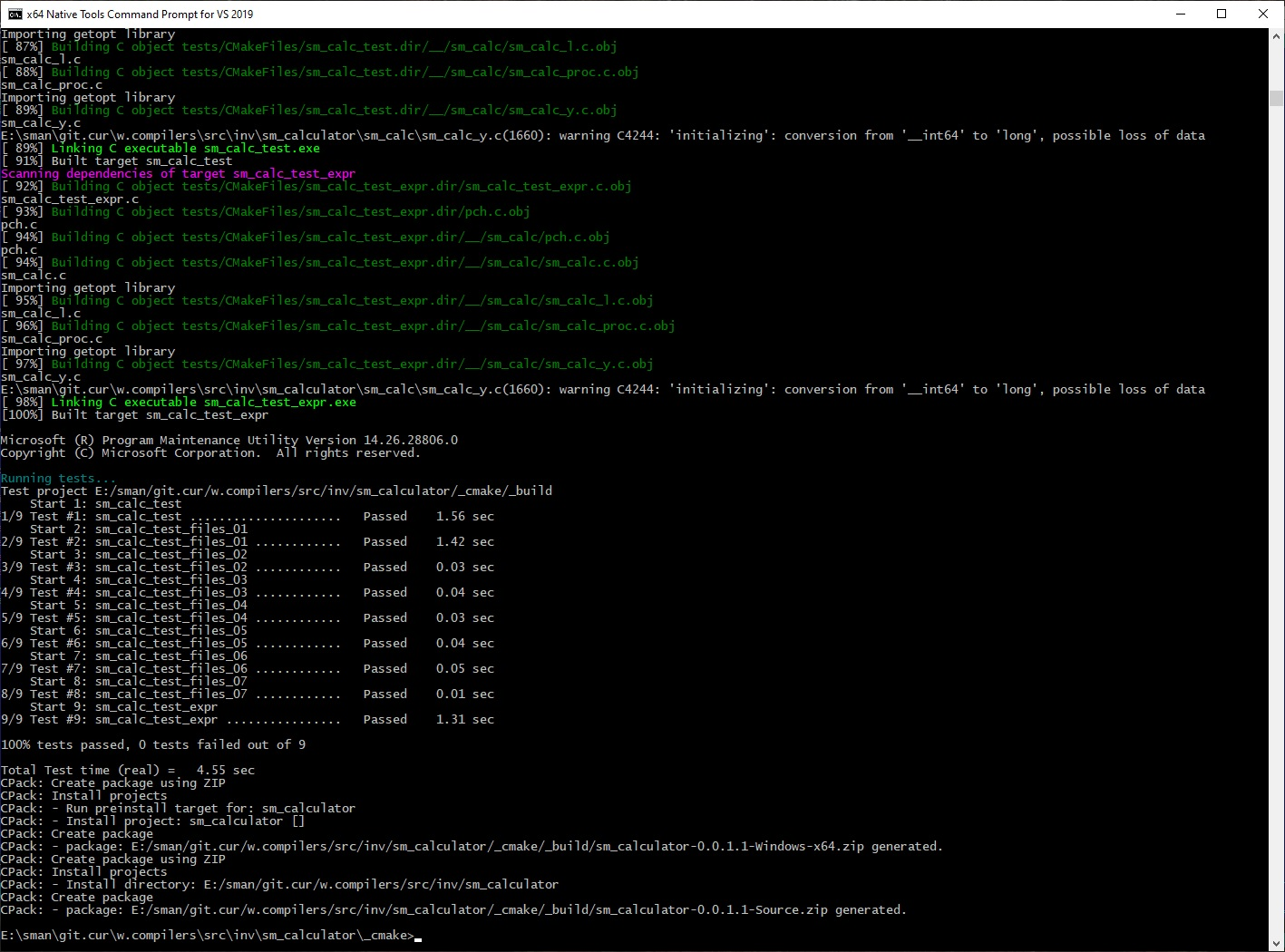Build of the sm_calculator (SM Calculator) solution 0.0.1.1 (Linux):
—————-

Here is a build screenshot, using
`/_cmake/cmake_build.sh`
See Install.txt for more details about the way of a build of the solution, using `/_cmake/cmake_build.sh`.

Build of the sm_calculator (SM Calculator) solution 0.0.1.1 includes following steps:

• creating of the subdirectory /_cmake/_build
• `cmake -G "Unix Makefiles" -DCMAKE_BUILD_TYPE="Release" "../..""` – generating of necessary project/build files, including Makefile for the Make tool
• `make clean` – clean target in Makefile (generated by CMake)
• `make all test` – build of the solution and launching unit tests
• `cpack -G "TGZ"` – generating pack archive, that contains binaries (.tar.gz)
• `cpack -G "TGZ" --config CPackSourceConfig.cmake` – generating pack archive, that contains sources (.tar.gz)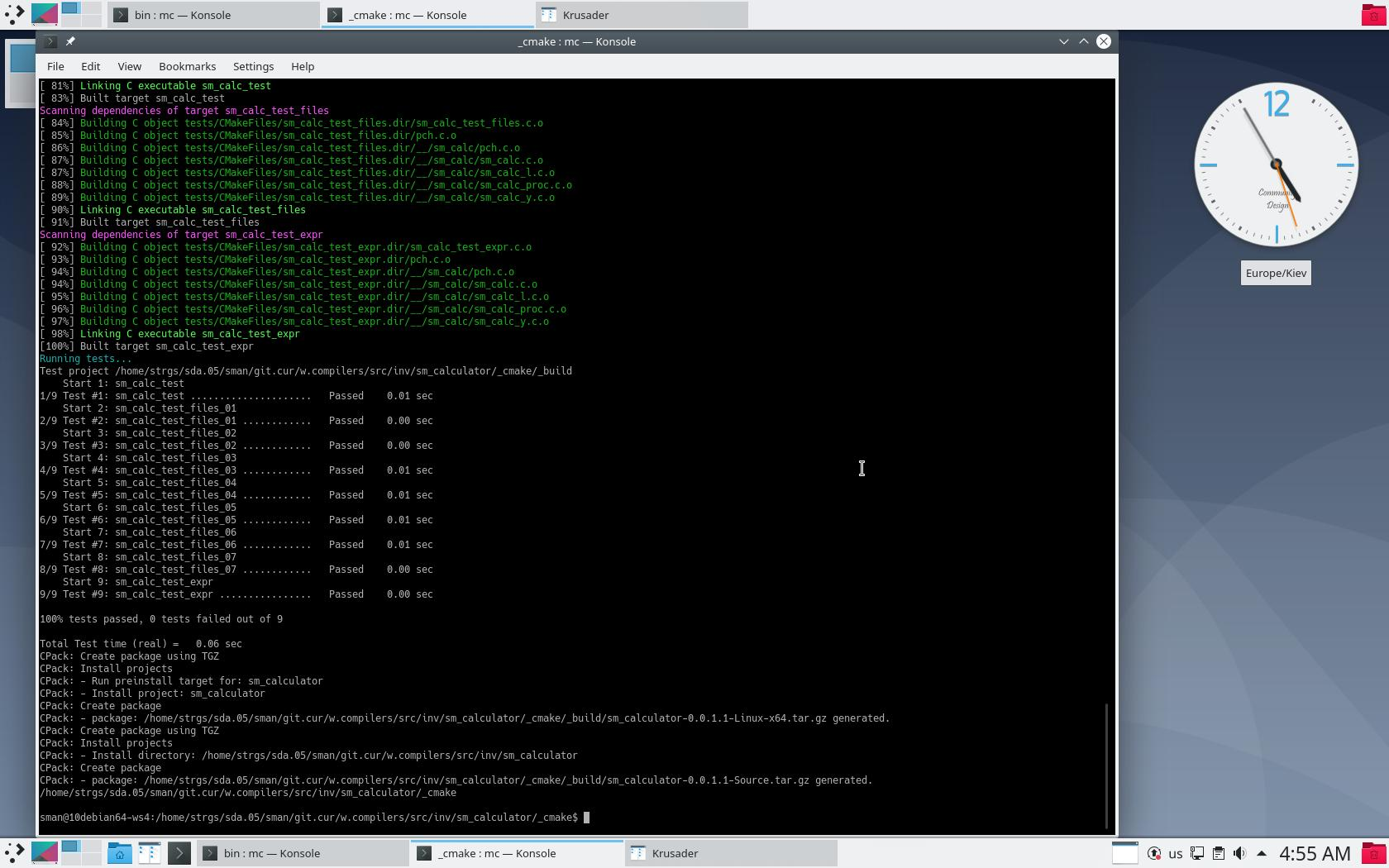—————-

# Multi-Platform Open-Source sm_calculator (SM Calculator) tool 0.0.1.1

Multi-Platform Open-Source sm_calculator (SM Calculator) tool 0.0.1.1 has been released.

Supported platforms:

• Windows (x86, x86_64)
• Linux (x86, x86_64)
• Examples, the suite of sm_calc_test.in.XX.txt and sm_calc_test.out.XX.txt (also you can find the suite of sm_calc_test.in.XX.txt and sm_calc_test.out.XX.txt in directory /tests in sources):
Examples

—————-

GPG Public Key (SManSoft ECDSA Key) <info@smansoft.com> has been published here: http://smansoft.com/gpg/smansoft.pub.asc.
SM Calculator – 0.0.1.1 contains suite of [some_install_file].asc files.
`gpg --import ./smansoft.pub.asc`
for importing of key and
`gpg --verify ./[some_install_file].asc ./[some_install_file]`
or
`gpg --verify ./[some_install_file].asc`
for verification of files, published on this site.
Public GPG ECDSA Key can be imported, using
`gpg --keyserver hkp://pgp.mit.edu --recv-keys A408B0FCFD774649`
too.

Features of the sm_calculator (SM Calculator) tool 0.0.1.1:
—————-

• Multi-platform application: there are builds for Windows 64/32 (.zip) and Linux 64/32 (.tar.gz);
• Various types of values (64 bits integers, 64 bits floats, strings);
• Various float constants (pi, exp,…);
• Solving of mathematical expressions, using basic arithmetic operators;
• Solving of mathematical expressions, using bracket delimiters ‘(‘ and ‘)’ (in one expression) and delimiters ‘;’, ‘\n’;
• Solving using suite of mathematical (including trigonometric, logarithm, exponentiation) functions;
• Solving using bitwise functions and operators;
• Various types of entering and output formats of integers (decimal, octal, hexadecimal, binary formats);
• Various values of the unit for measuring of angles (radians, degrees, gradians), which are used by trigonometric functions;
• Support of output properties (precision) of float values;
• The suite of converting functions (various values of the unit for measuring of angles, various types of entering and output formats of integers);
• the suite of commands, which provide setup of the configuration of SM Calculator;
• Several modes of execution (processing of files, processing of expressions, obtained via command line, processing of expressions in interactive mode, using standard input/output devices);
• Help (command: help[;] or command-line option: -h, –help);

SM Calculator tool supports following modes of execution:
—————-

• processing of the file, that contains expression(s);
• processing of command-line expression(s);
• interactive mode (user enters expression(s), using standard input device/console).

A user can use the same expressions in all these modes (processing of the file,
processing of command-line expression(s), interactive mode). I.e. SM Calculator tool supports the same lexemes and grammar rules in all modes.

Limitations/disadvantages of the sm_calculator (SM Calculator) tool 0.0.1.1:
—————-

• sm_calculator (SM Calculator) tool 0.0.1.1 (after launching) creates/opens log file: ./sm_calc.log  in the current directory. I.e. user should take into account, that ./sm_calc.log  will be created/updated in the current directory;
• sm_calculator (SM Calculator) tool 0.0.1.1  writes full log with detailed info without filtering of log messages by log level;
• if you use Linux and launches sm_calculator from another directory, where sm_calc module is deployed (bin/sm-calc), you should copy file /lib/libgetopt_lib.so to one of the directories /usr/local/lib, /usr/lib or use follow launch of sm_calc:
`export LD_LIBRARY_PATH=/home/some_user/bin/sm_calculator/lib; /home/some_user/bin/sm_calculator/bin/sm_calc`

These limitations/disadvantages of the sm_calculator (SM Calculator) tool 0.0.1.1 will be fixed/resolved in the next version.

Structure of binary archive (.zip or .tar.gz):
—————-

• `.zip (Windows):`
```    bin\
sm_calc.exe
share\smansoft\doc\
HELP
```
• `.tar.gz (Linux):`
```    bin/
sm_calc
lib/
libgetopt_lib.so
share/smansoft/doc/
HELP
```

Execution:
—————-
`sm_calc[.exe]`
without parameters launches SM Calculator in interactive mode (default mode):

``````sm_calc 0.0.1.1 Copyright (c) 2020 SManSoft
SM Calculator
``` ```    Interactive processing:
In >>``````

User can run:
`sm_calc[.exe] -h`
or
`sm_calc[.exe] --help`
to get help output – description of command-line parameters.

Using these options a user can launch SM Calculator in various modes of execution and tune start values of the current configuration of SM Calculator (command: config[;]):

```-h, --help -i, --interactive -f<infile>, --in_file=<infile> -o<ofile>, --out_file=<ofile> -x<expression_value>, --expression=<expression_value> -p<precision_value>, --f_precision=<precision_value> -n<i_format_value>, --i_format=<i_format_value> -a<unit_value>, --a_unit=<unit_value>```

Lexemes of SM Calculator expressions:
—————-

Available keywords:
`f_precision i_format a_unit pi exp gamma phi rad deg grad grad2rad rad2grad rad2deg deg2rad grad2deg deg2grad dec oct hex bin dec2oct dec2hex dec2bin oct2dec hex2dec bin2dec sin cos tan asin acos atan ln lg log inv sqrt rand pow pow_exp^ pow_10^ pow_2^ pow_^2 abs mod mod2 and or not nand nor xor config help echo quit exit`

Available operators:
`+ - * / = ! <a< >a> <l< >l> <c< >c>`

Available delimiters:
`, ( ) ; \n (new line)`

Commands (from available keywords):
`f_precision i_format a_unit dec oct hex bin rad deg grad rand config help quit exit`

Constants (from available keywords):
`pi exp gamma phi`

Output functions:
`echo`

Converting functions (from available keywords):
`grad2rad rad2grad rad2deg deg2rad grad2deg deg2grad dec2oct dec2hex dec2bin oct2dec hex2dec bin2dec`

Trigonometric functions (from available keywords):
`sin cos tan asin acos atan`

Logarithm functions (from available keywords):
`ln lg log`

Exponentiation functions:
`pow pow_exp^ pow_10^ pow_2^ pow_^2`

Bit functions (from available keywords):
`and or not nand nor xor`

Bit operators (from available operators):
`<a< >a> <l< >l> <c< >c>`

Base syntax of commands:
—————-
`command[;]`
`command=arg[;]`

Base syntax of functions:
—————-
`function (arg1[,arg2])[;]`

Base syntax of operators:
—————-
`arg1 operator arg1[;]`
`operator arg[;]`

Operator assign (‘=’) can be used only in commands (f_precision, i_format, a_unit).

Types of values:
—————-

• Integer data (long long type – 64 bits);
• Float data (long double type – 64 bits);
• Strings (max length of string 1021 ASCII symbols);

Integer data:
—————-
Length of Integer data – 64 bits (including sign), i.e.
max integer value == 2^63-1;
min integer value == -(2^63-1);
Integer value can be entered and output in follow formats:

• decimal (‘dec‘);
• octal (‘oct‘);
• binary (‘bin‘);

The output format of integer value can be defined by command:
`i_format = <dec|hex|oct|bin>[;]`
A user can use shorter way for defining of the output format of an integer value, using commands:
`dec[;]`
`hex[;]`
`oct[;]`
`bin[;]`

The input format of an integer is defined by following rules:

• if a user enters integer value in octal format, he should use as least one lead ‘0’ symbol (for example: ‘0712377’, ‘-0712377’);
• if user enters integer value in hexadecimal format, he should use as lead ‘0x’ symbols (for example: ‘0x394ff’, ‘0xfffffffffffc6b01’);
• if user enters integer value in binary format, he should use as lead ‘b’ symbol (for example:  ‘b00111001010011111111’‘b1111111111111111111111111111111111111111111111000110101100000001’);
• if user enters integer value in decimal format, he shouldn’t use any additional symbols (for example: ‘129077’‘-129077’);

A user should take into account, that size of integer value is 64 bits (including sign), during entering value in various formats;

A user can enter negative values (in octal and hexadecimal) using as sign ‘-‘
as two’s complement format (see examples in the file ReadMe.txt):
-0712377 (oct) or 01777777777777777065401 (oct) == -234751 (dec)
-0x394ff (hex) or 0xfffffffffffc6b01 (hex) == -234751 (dec)

If user need to get current output format of integer values, he needs to use command:
`i_format[;]`

SM Calculator tool supports the suite of functions, which provide converting of various formats of integer values, whatever defined current output format of integer values:
`dec2oct dec2hex dec2bin oct2dec hex2dec bin2dec`.

Float data (long double – 64 bits):
—————-
The output format of float values always defined in decimal format. User can tune the precision of float value output, using the command:
`f_precision = N[;]`
, where N = [0,20]|exp, i.e. maximum precision of output of float value is ’20’.

Also, N can be defined as ‘exp’. In this case, the float value will be printed in an exponential format.

The input format of float values always defined in decimal format. A user can enter values as in floating form format as in exponential format.

If a user needs to get current output current precision of float, he needs to use the command:
`f_precision[;]`.

Strings:
—————-
User should enter string value, using the following format:
`"string_value" | 'string_value'`.
Max length of the string – 1023 ASCII symbols with quotes(‘ or “)
or 1021 ASCII symbols without quotes(‘ or “).

String values aren’t used during calculations but could be used for the formatting of outputs in file processing, using the function
`echo`.

Default angle unit configuration:
—————-
SM calculator supports some suite of trigonometrical functions:
`sin cos tan asin acos atan`.
Also, SM calculator supports follow units for measuring of angles:

• degrees(‘deg‘)

SM Calculator tool contains the current configuration of the unit for measuring of angles. This parameter defines, which unit for measuring of angles will be used by trigonometric functions, i.e. if the current configuration of the unit for measuring of angles is radians, a user should use radians as parameters of trigonometric functions: sin(3.1415926536) or sin(pi), cos(1.5707963268) or cos(pi/2.0), if the current configuration of the unit for measuring of angles is degrees, a user should use degrees as parameters of trigonometric functions: sin(180.0), cos(90), same issue with the usage of gradians.
The current configuration of the unit for measuring of angles can be defined by the following command:
`a_unit=<deg|rad|grad>[;]`

Also use can setup current configuration of the unit for measuring of angles, just using following commands:
`deg[;]`
`rad[;]`
`grad[;]`

User can get current configuration of the unit for measuring of angles using the command:
`a_unit[;]`

SM Calculator tool supports the suite of functions, which provide converting of the various unit for measuring of angles, whatever defined current configuration of the unit for measuring:

`grad2rad rad2grad rad2deg deg2rad grad2deg deg2grad`

Current configuration:
—————-
A user always can get current configuration, using the command:
`config[;]`
The result of the command config[;]:
```Current Configuration : --------------------------------------------- Current double precision : EXPONENTIAL; Current output format of integers : dec; Unit of measurement of an angle : rad;```

Constants:
—————-
SM Calculator supports follow float (64 bits) constants:
`pi`
`exp`
`gamma`
`phi`

Constants return float values. They can be used in expressions as arguments of functions and operators.

Examples:
—————-
User can find here suite of files which contain suite examples of usage of operators, functions, commands,
constants, various types of data:

Examples

 Examples of expressions: Results of execution of expressions: sm_calc_test.in.01.txt sm_calc_test.out.01.txt sm_calc_test.in.02.txt sm_calc_test.out.02.txt sm_calc_test.in.03.txt sm_calc_test.out.03.txt sm_calc_test.in.04.txt sm_calc_test.out.04.txt sm_calc_test.in.05.txt sm_calc_test.out.05.txt sm_calc_test.in.06.txt sm_calc_test.out.06.txt

—————-

Help.txt:
—————-
The short reference of commands, constants, functions, operators of the sm_calculator (SM Calculator) tool 0.0.1.1 is published here:
Help.txt

Demo of the sm_calculator (SM Calculator) tool 0.0.1.1 (Windows):
—————-

Here are the results of the execution of the sm_calculator (SM Calculator) tool 0.0.1.1 on Microsoft Windows 10 x64.

The sm_calculator (SM Calculator) tool 0.0.1.1 has been launched 4 times:

1. `sm_calc --help`
2. `sm_calc --expression="pow_^2(sin(pi/2))+pow_^2(cos(pi/2));"`
3. `sm_calc --in_file="./sm_calc_test.in.01.txt" --out_file="./sm_calc_test.out.01.txt"`
4. `sm_calc`

Results of execution (log file and screenshots) below:
sm_calc.log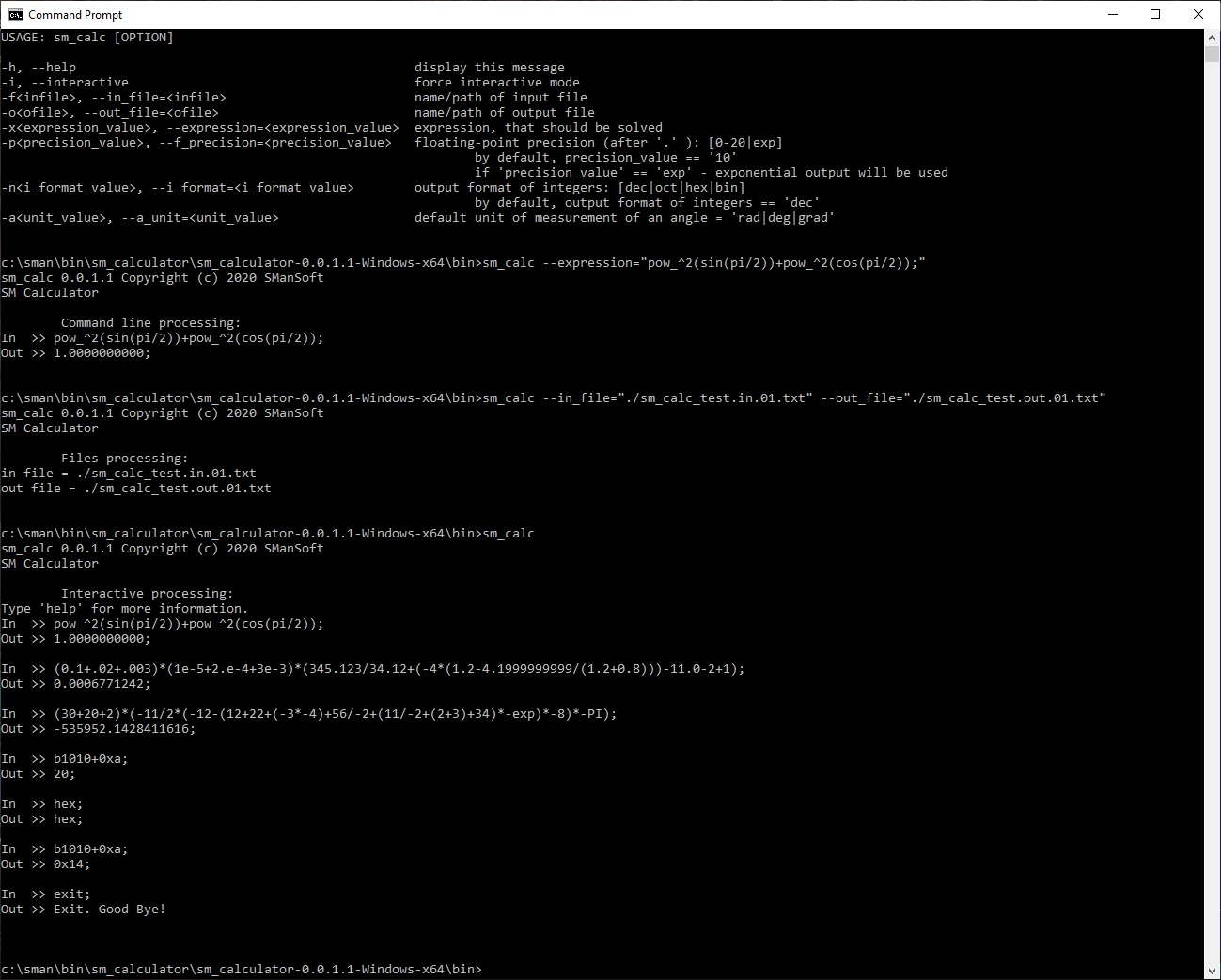Demo of the sm_calculator (SM Calculator) tool 0.0.1.1 (Linux):
—————-

Here are the results of the execution of the sm_calculator (SM Calculator) tool 0.0.1.1 on Linux Debian 10 (Buster) x64.

The sm_calculator (SM Calculator) tool 0.0.1.1 has been launched 4 times:

1. `sm_calc --help`
2. `sm_calc --expression="pow_^2(sin(pi/2))+pow_^2(cos(pi/2));"`
3. `sm_calc --in_file="./sm_calc_test.in.02.txt" --out_file="./sm_calc_test.out.02.txt"`
4. `sm_calc`

Results of execution (log file and screenshots) below:
sm_calc.log